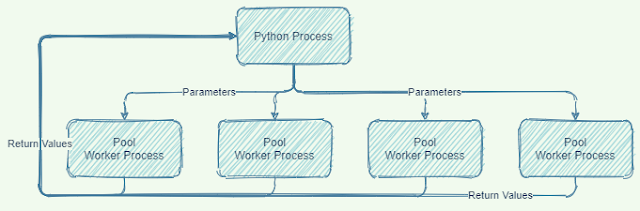### Python multiprocessing.Pool improvement examples in Donor's Choice data

We're going to walk through a couple places where simple Python parallelization created big performance improvements using the Elasticsearch Donor's Choice prep scripts on GitHub .  This is a clone of the Elasticsearch GitHub repository of the same name.

My rule of thumb for this particular processing section was that I would only move to parallel execution if execution time was reduced within 80% of the number of extra processors. So with 8 cores I wanted to get at least 6 times performance.

## Python Multiprocessing Parallel Execution

Python is essentially single threaded in many situations.  Thread based parallelization is pretty much only useful for I/O bound situations like web requests where the threads are idle most of the time. Compute bound parallel execution is usually multi-processor with work being fed off to essentially different programs.No Shared State
Each processing unit in multi-processing parallel execution runs in its own address space.  Data must be copied to the worker processes and results must be copied back to  the main process.  This overhead can constrain the cases where multiprocessing is worth doing.

## Video

### Text Wrapping Comment

Some of the code samples are wider than the blog frame.  I left the wrap that way because all the important parts of this are on the left part of each source code line.

## Is This I/O routine worth making Parallel?

This code loads three data files.  It looks like a great candidate for parallel execution.
start = perf_counter()
end = perf_counter()
print(end - start)

It turns out that the size of one of the data sets is 3 times the size of the other two combined.  This means that It is only possible to achieve a 25% reduction by loading the files in parallel.  IMO wasn't worth the complexity for this simple project.

## How about Grouping the data by ProjectId?

This section of code iterates across the result of a groupBy operation. resources contains columns for 8 different categories.

resources_grouped_by_projectid = resources.groupby('projectid')

# try and set this up for parallel operations later
def do_concat(one_group_by):
return one_group_by.apply(lambda x: concatdf(x))

for a in resources.columns.values:
# Iterate across the DataFrameGroupBy operating on one SeriesGroupBy at a time
print("column "+ a)
concat_resource[a]=do_concat(resources_grouped_by_projectid[a])
# print(concat_resource[a])

The operation takes 900 seconds across all iterations. It feels like we cold get this down to under 200 seconds if we processed each of the 8 in parallel. We have millions of records to process.

The following code executes the iterator in parallel reducing the time about 75% to 170 seconds. pool.starmap accepts parameters that split up among the workers. It splits the passed in parameter so that each pool worker gets a 1/num_workers sized portion.  It then merges the result from each pool thread and returns them.
resources_grouped_by_projectid = resources.groupby('projectid')

# return a tuple we can assign
def do_concat_by_index(index):
print("starting : "+index)
return (index, resources_grouped_by_projectid[index].apply(lambda x: concatdf(x)))

indexes = resources.columns.values
print ('Manipulating : {}'.format(indexes))
# pool size could be 8 the number in of tasks we need
with Pool(10as pool:
our_result = pool.starmap(do_concat_by_index, zip(indexes))

# move the results from the return list into concat_result
for one_result in our_result:
# print('one result index is {} '.format(one_result))
concat_resource[one_result]=one_result

## A series of atomic operations across the entire data set

The next block executes reformatting steps against various attributes. Each line transforms a single field field on each row of the data set. Each transformation runs against the entire data set.  The reformatting of the attributes of a single record have no impact on the operations being done on other records .  This took 1450 seconds on a 2Ghz machine.
data['project_date_expiration'] = data['project_date_expiration'].map(lambda x: str_to_iso(x));
data['project_date_posted'] = data['project_date_posted'].map(lambda x: str_to_iso(x))
data['project_date_thank_you_packet_mailed'] = data['project_date_thank_you_packet_mailed'].map(lambda x: str_to_iso(x))
data['project_date_completed'] = data['project_date_completed'].map(lambda x: str_to_iso(x))
data['donation_timestamp'] = data['donation_timestamp'].map(lambda x: str_to_iso(x))

# Create location field that combines lat/lon information
data['project_location'] = data[['project_school_longitude','project_school_latitude']].values.tolist()
del(data['project_school_latitude'])  # delete latitude field
del(data['project_school_longitude']) # delete longitude

The parallel version splits the input data frame into segments, one for each processor in the Pool. Each segment is fed to its own process. This means all the data gets copied to worker processes. All the same data , with modifications, is copied back. This took 380 seconds , about a 70% savings.  It is possible that the copy-in/copy-out overhead is high enough to get this low multiple improvement.
def do_date_fix(some_data):
#print(some_data.describe())
some_data['project_date_expiration'] = some_data['project_date_expiration'].map(lambda x: str_to_iso(x));
some_data['project_date_posted'] = some_data['project_date_posted'].map(lambda x: str_to_iso(x))
some_data['project_date_thank_you_packet_mailed'] = some_data['project_date_thank_you_packet_mailed'].map(lambda x: str_to_iso(x))
some_data['project_date_completed'] = some_data['project_date_completed'].map(lambda x: str_to_iso(x))
some_data['donation_timestamp'] = some_data['donation_timestamp'].map(lambda x: str_to_iso(x))

# Create location field that combines lat/lon information
some_data['project_location'] = some_data[['project_school_longitude','project_school_latitude']].values.tolist()
del(some_data['project_school_latitude'])  # delete latitude field
del(some_data['project_school_longitude']) # delete longitude
return some_data

num_workers = 8
data_split = np.array_split(data,num_workers)
with Pool(num_workers) as pool:
fixed_dates = pool.map(do_date_fix,data_split)
data = pd.concat(fixed_dates)

## Elasticsearch

The Elasticsearch Python library contains single threaded and parallel processing versions of it's indexing methods. Making that one line change saved over 50%.  I don't know why the savings isn't higher.
if not success:
print('A document failed:', info)

## Conclusion

Implementing two parallel changes plus the Elasticsearch parallel load API knocked 105 minutes off the wall time for preparation and indexing on a 2Ghz machine
• 920sec --> 169sec  Grouping
• 1450sec --> 380sec Date Manipulation
• 8223sec --> 3686sec Elasticsearch Indexing
6358 seconds saved or 105 minutes saved

You can achieve big savings on large data operations if they are mostly independent of each other. The extra code and data management complexity may or may not be worth it depending on how time sensitive you are and how much complexity you wanted to manage.